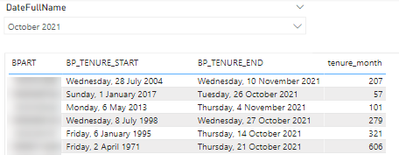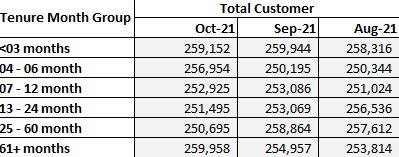cancel
Showing results for
Did you mean:Anonymous
Not applicable

## Group tenure months and count the total

Hi All,

I'll explain the data first:

I have a data set with

- customer number (BPART)

- start date of tenure

- end date of tenure

In order to report the total count of customer for each month, I have a relationship of many to many through a bridge to a Date dimensionSo for instance, if the customer tenure starts in "jan2020" and ends in "December2021", and i am reporting for the month of "March2020", then I can count that customer as active for that month, and so on..

I can calculate the tenure in months for each customer using dax, and when I change the DATE, then the tenure changes acordingly

``tenure_month = DATEDIFF(ENDOFMONTH(BP_TENURE_STAGE_BRIDGE[BP_TENURE_START]),MAX(DIMDATE[DateFullName]),MONTH)``What I need to do is to group this "tenure month" and count the total customers for each group, using the following grouping

• < 3 months
• 4-6 months
• 7-12 months
• 13-24 months
• 25-60 months
• +61 months

This has to be dinamyc, because when I change the reporting period (from the Date Dimension), the tenure for some customer may move from one bucket of tenure into the other one.

So it would have to look something like this:I imagine that this has to be achieved by creating a virtual table that summarises the values, but I cant figure out how to do it.

Due to sensitive data I cant share the PBI file

Thank you very much, and let me know if any extra clarification is needed

2 REPLIES 2Super User

@Anonymous , I have used these formula in HR with a Date table with some changes they should work in this case

Method 1

Employees = COUNT(Emp[Employee Id ])
Hire = CALCULATE([Employees], USERELATIONSHIP('Date'[Date], Emp[Start Date]))
Teminated = CALCULATE([Employees], USERELATIONSHIP('Date'[Date], Emp[End Date]), not (ISBLANK(Emp[End Date])))

Total Emp = CALCULATE( Countx(FILTER(Emp, Emp[Start Date] <= Max('Date'[Date]) && (ISBLANK(Emp[End Date]) || Emp[End Date] >Max('Date'[Date]))),[Employees]) , CROSSFILTER('Date'[Date],Emp[Start Date],None))

Method 2

Cumm Hire = CALCULATE([Hire], FILTER(ALL('Date') , 'Date'[Date] <= max('Date'[Date])))
Cumm Termination = CALCULATE([Teminated], FILTER(ALL('Date'), 'Date'[Date] <= Max('Date'[Date])))
Current Employee = [Cumm Hire] -[Cumm Termination]

Date table is joined with a start date as active and end date with inactive join

https://community.powerbi.com/t5/Community-Blog/HR-Analytics-Active-Employee-Hire-and-Termination-trend/ba-p/882970Anonymous
Not applicable

Hi Mate, this looks great, but unfortunately it doesnt answer my main pain point whihc is creating virtually these bucket of tenure. I need to be able to group them and count the total by group, and that total has to change along with the customer moving onto diff buckets when I change the date